# Propellant Selection¶

Before looking at stage performance, selecting a bipropellant combination for an application can have many considerations.:

```Historical Experience
Stage/Vehicle Cost
Propellant Cost
Production Run Size
Propellant Handling Requirements
Propellant Production Sources
Propellant Toxicity
Hypergolicity
Common Propellant Liquidus Range
Propellant Vapor Pressure or Freezing Limits
Launch Hold-Time Limits
Insulation Requirements
Insulation Purge Requirements
Cleanliness Requirements
Material Compatibility Requirements
Propellant Line Filling Procedures
Propellant Line Velocity Limits
Propellant Storage Life
Storage Conditioning Requirements
Propellant Venting
etc.
```

The list of candidate propellants can usually be pruned down to just a few that satisfy the basic application requirements above.

For most applications, the performance goal is usually a combination of:

```Fit Into a Volume
Weigh Less Than a Limit
Deliver a Delta Velocity
Deliver an Acceleration
```

The best way to evaluate performance differences between propellants that survive the initial screening is to have engineering teams generate detailed designs for each of the propellants and pick the best detailed design.

In practice, that is rarely a viable option due to the cost and schedule of generating detailed designs.

## Rough Screening¶

The most common tool for simplified performance screening is a chart of propellant `density impulse` (Isp * bulk propellant density).

It is very clear that high Isp is good and high density is good so it’s natural to assume that the linear combination of Isp and density must be good also. Many companies publish charts of density impulse such as this TRW Propellant Performance Summary

The units of density impulse can vary between charts, however the choice of `specific gravity` for density and `sec` for Isp seems to keep numbers in more recognizable ranges. Most charts use an X axis of `Mixture Ratio` and a combined Y axis of `Isp` and `Density Impulse`.

The same information can also be displayed on a chart like the one below. Here the X axis is `Specific Gravity (g/ml)` and the Y axis is `Isp`. On that chart, contours of `Density Impulse` can be shown.

The bipropellant Isp curves correspond to CEA equilibrium Isp vacuum at the given chamber pressure and area ratio. Recall that real engine Isp will be derived from a curve between equilibrium and frozen as shown in LOX/LH2 Performance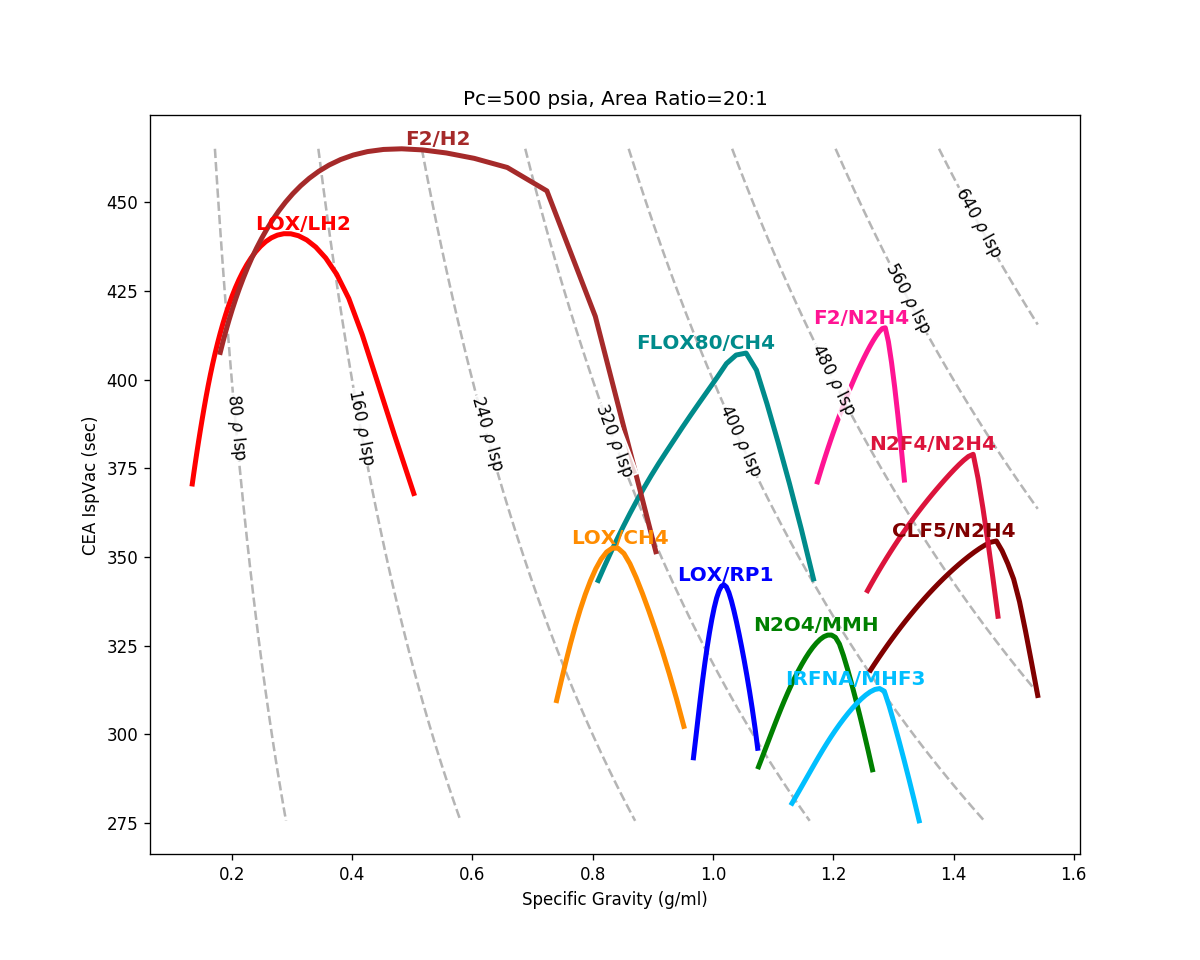Click Image to View Fill Size

The script that created this chart is.

```from rocketcea.biprop_utils.rho_isp_plot_obj import RhoIspPlot
rp = RhoIspPlot()
rp.show()
```

Looking at the chart above, it says that if Isp is most important, then only use F2/H2 or perhaps LOX/LH2 if Fluorine is rejected.

If density impulse is most important, then only use N2F4/N2H4 or perhaps LOX/RP1 if LOX is the chosen oxidizer.

## Stage Density¶

In order to help bring some order into the decision making process, it is possible to introduce a new parameter called Stage Density.:

```Stage Density = (Stage Inert Mass) / (Stage Propellant Volume)
```

If we assume that Stage inert mass only depends on tank volume. Then no matter which bipropellant combination we pour into the stage or in whatever mixture ratio we pour it, all of the stage performance parameters can be calculated.

This allows us to take a reference stage design and evaluate its performance when using any propellant combination.

The quality of this assumption will certainly vary between propellant combinations. The performance of some propellants will be helped and others will be hurt, however, a review of any results can take those differences into account.

## Volume Limit¶

A common requirement on a stage is to meet a volume limit. This is the easiest application of the stage density idea. Using a fixed stage design with a fixed propellant volume, simply pour in that volume of various propellants and evaluate their performance.

As an example, say that the first stage of the Saturn V was designed to have a maximum propellant volume of 75500 cubic feet (its actual volume). For every point on the specific gravity vs Isp chart, lines of constant stage delta velocity and stage gross liftoff weight (GLOW) can be drawn by assuming that the inert mass of the stage does not change regardless of the propellant used in the stage.

Every point on the chart below has the same volume.

Notice that for much of the chart, that the lines of constant density impulse closely match the lines of constant delta v. For these conditions, it looks like LOX/RP1 was a good choice for the Saturn V first stage.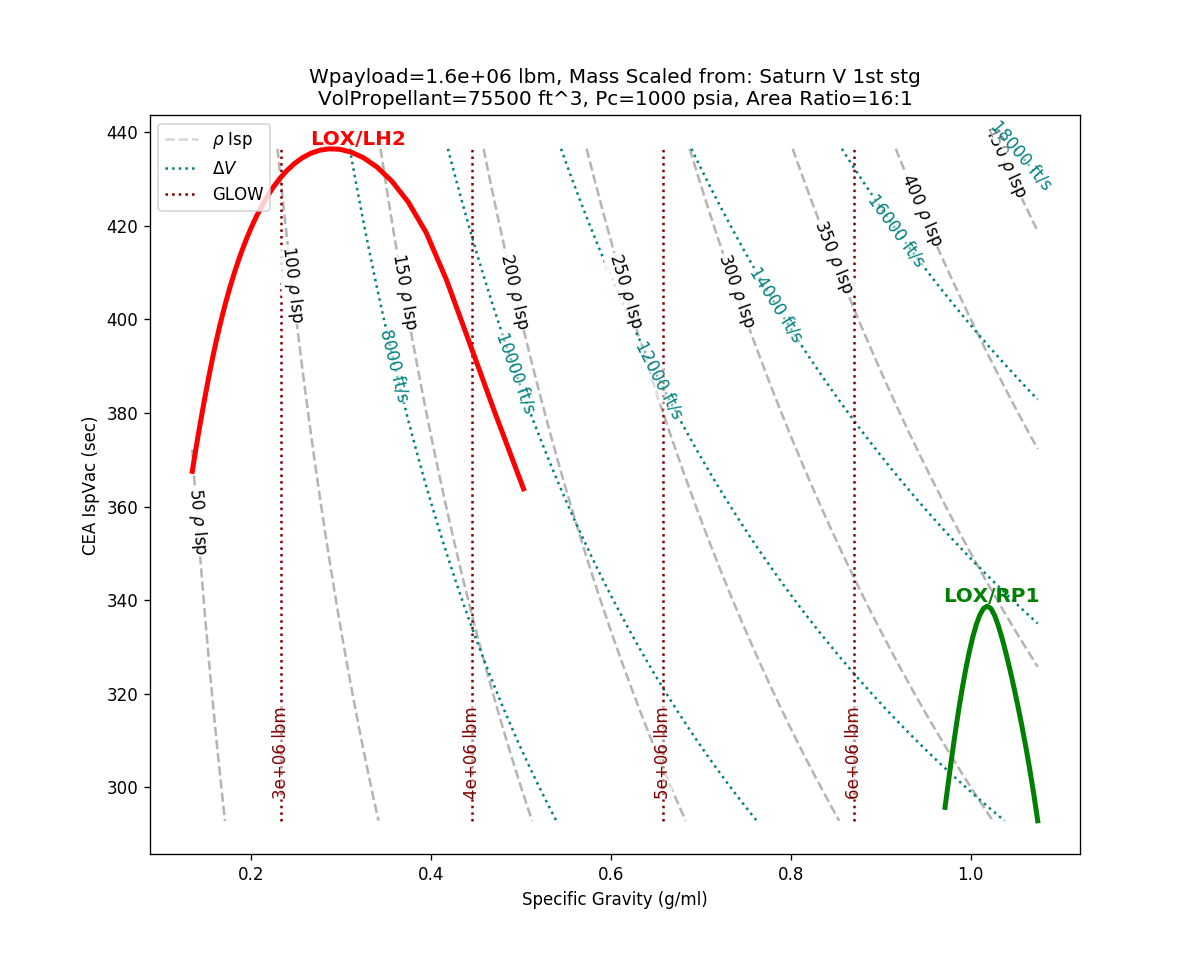Click Image to View Fill Size

The script that created this chart is.

```from rocketcea.biprop_utils.rho_isp_plot_obj import RhoIspPlot
from rocketcea.biprop_utils.veh_stage_obj import ReferenceStage

rp = RhoIspPlot(bipropL=[('LOX','LH2'), ('LOX','RP1')], Pc=1000., eps=16)

stg_obj = ReferenceStage( WtPayload=1600000.0, volCuInRef=75500.0*1728, WtInertRef=300000.0,
Name='Saturn V 1st stg')

label_frac_posD={'GLOW':0.1, 'CubicFt':.4, 'MassFrac':.5},
plot_param_valD={'DeltaV':[8000,10000,12000,14000,16000,18000],
'MassFrac':[0.86,0.88,0.9,0.92,0.94,0.95],
'GLOW':[3e6, 4e6, 5e6, 6e6, 7e6, 8e6, 9e6]    },
plot_paramL=['DeltaV','GLOW'], num_ticks=6)
rp.show()
```

## Delta V Requirement¶

Another common requirement for a stage is to deliver a certain delta velocity. the use of stage density in this scenario requires that a function for stage density be derived. The chart below scales the actual Saturn V stage from an historical chart of stage density. Another good approach is to estimate stage designs at points of interest on the chart and scale between them.

Every point on the chart below delivers the same delta velocity.

What this chart says is that a LOX/LH2 first stage would weigh a bit less than the existing LOX/RP1 stage, but it would be twice the size.

It is interesting to note here also that the lines of density impulse and those of propellant volume track closely together in an inverse relationship.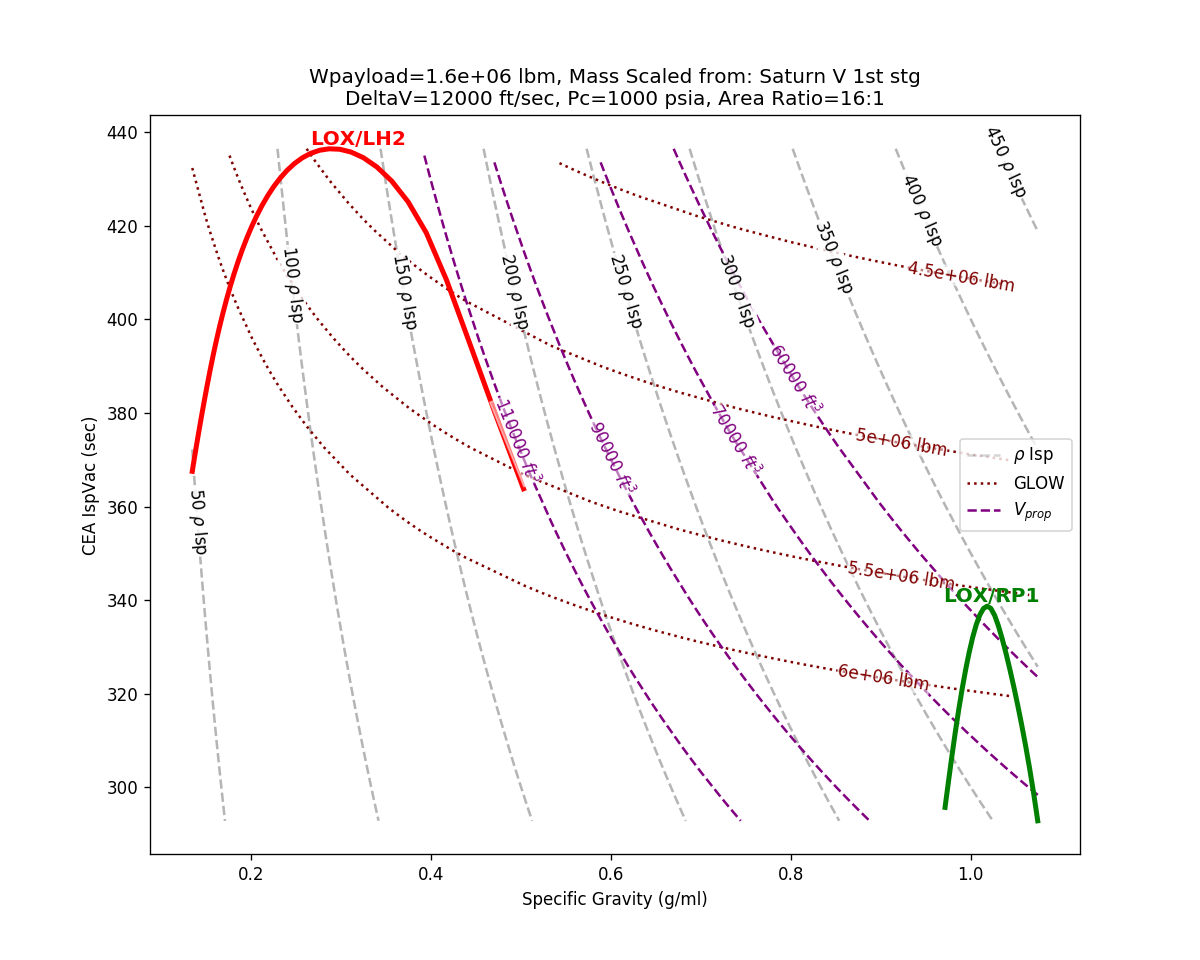Click Image to View Fill Size

The script that created this chart is.

```from rocketcea.biprop_utils.rho_isp_plot_obj import RhoIspPlot
from rocketcea.biprop_utils.veh_stage_obj import ReferenceStage

rp = RhoIspPlot(bipropL=[('LOX','LH2'), ('LOX','RP1')], Pc=1000., eps=16)

stg_obj = ReferenceStage( WtPayload=1600000.0, volCuInRef=75500.0*1728, WtInertRef=300000.0,
Name='Saturn V 1st stg')

label_frac_posD={'GLOW':0.9, 'CubicFt':.4, 'MassFrac':.8},
plot_param_valD={'GLOW':[6e6, 5.5e6, 5e6, 4.5e6],
'CubicFt':[60000, 70000, 90000, 110000, 13000]},
plot_paramL=['GLOW','CubicFt'], num_ticks=6)
rp.show()
```

## Mass Limit¶

A common requirement, especially for upper stages, is to minimize mass for a given delta v. The volume is not as important since upper stages are the smallest stages already. Using stage density to evaluate this situation again requires a function for stage density. The stage density function can be a scaling of a single design point or the interpolation between various design points.

The chart below is an evaluation of the LOX/LH2 third stage of the Saturn V.

Every point on the chart has the same initial mass. This means that the lower stages will deliver the same delta v for all points on the chart.

Given this, the chart shows that using LOX/LH2 for the third stage will deliver about 1000 ft/sec more than a LOX/RP1 or a LOX/CH4 third stage.

Note that in this case of `mass limit`, density impulse is a poor indicator of the best propellant combination.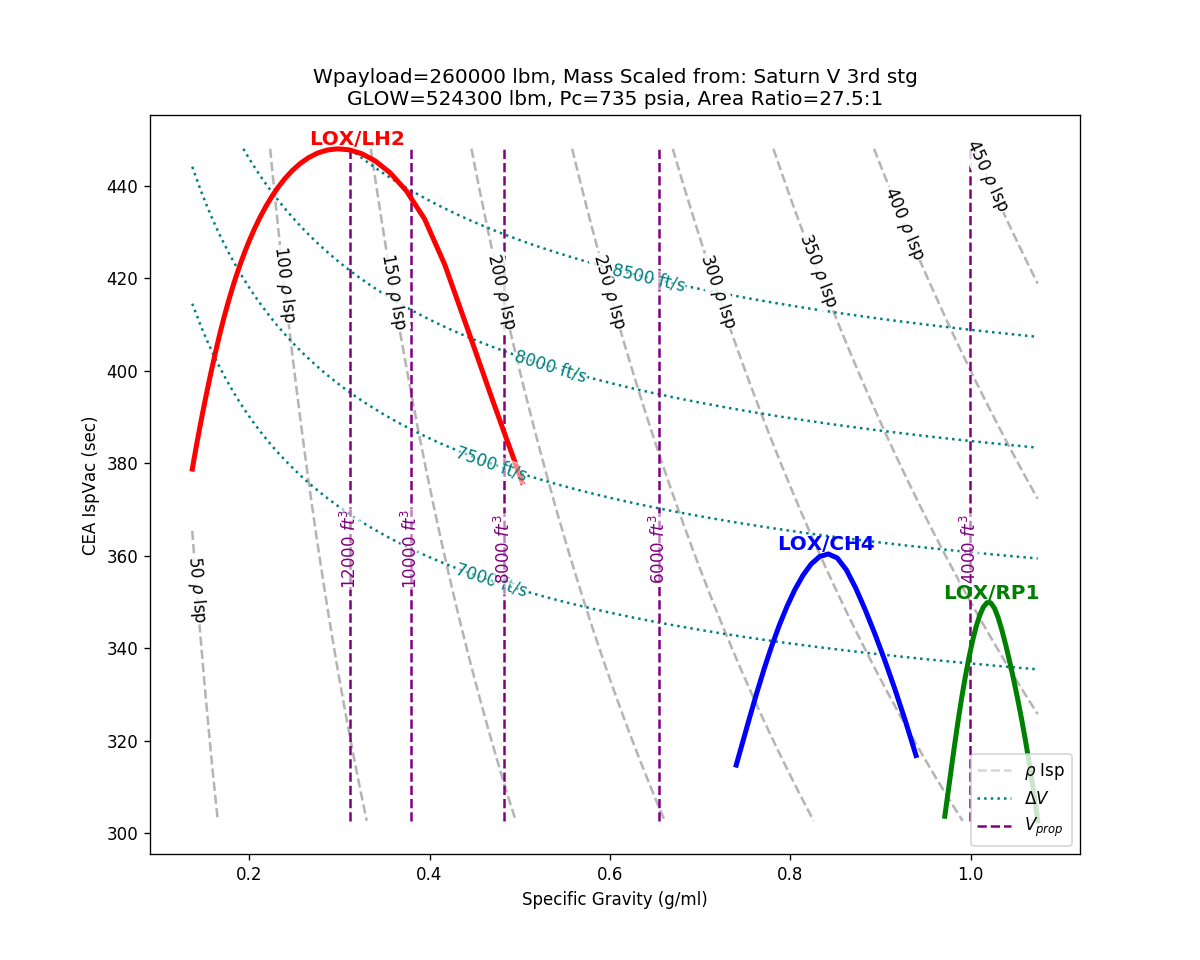Click Image to View Fill Size

The script that created this chart is.

```from rocketcea.biprop_utils.rho_isp_plot_obj import RhoIspPlot
from rocketcea.biprop_utils.veh_stage_obj import ReferenceStage

rp = RhoIspPlot(bipropL=[('LOX','LH2'), ('LOX','RP1'), ('LOX','CH4')], Pc=735., eps=27.5)

stg_obj = ReferenceStage( WtPayload=260000.0, volCuInRef=11180*1728, WtInertRef=29300.0,
Name='Saturn V 3rd stg')

label_frac_posD={ 'CubicFt':.4, 'DeltaV':.5},
plot_param_valD={ 'CubicFt':[4000, 6000, 8000, 10000, 12000],
'DeltaV' :[7000, 7500, 8000, 8500] },
plot_paramL=['DeltaV','CubicFt'], num_ticks=6)
rp.show()
```

## Monopropellants¶

Screening with monopropellants can also be done using `stage density`, however, some care must be taken in the `stage density function`.

Note also that monopropellants are only a single point on the specific gravity vs Isp curve.

The chart below compares different propellants being used in a divert vehicle.

Every point on the chart has the same initial mass.

Note that in this case with a `mass limit`, but a much lower delta velocity requirement, that density impulse is a better indicator of performance than it was for the Saturn stage 3 case, but only by luck, not based on system requirements.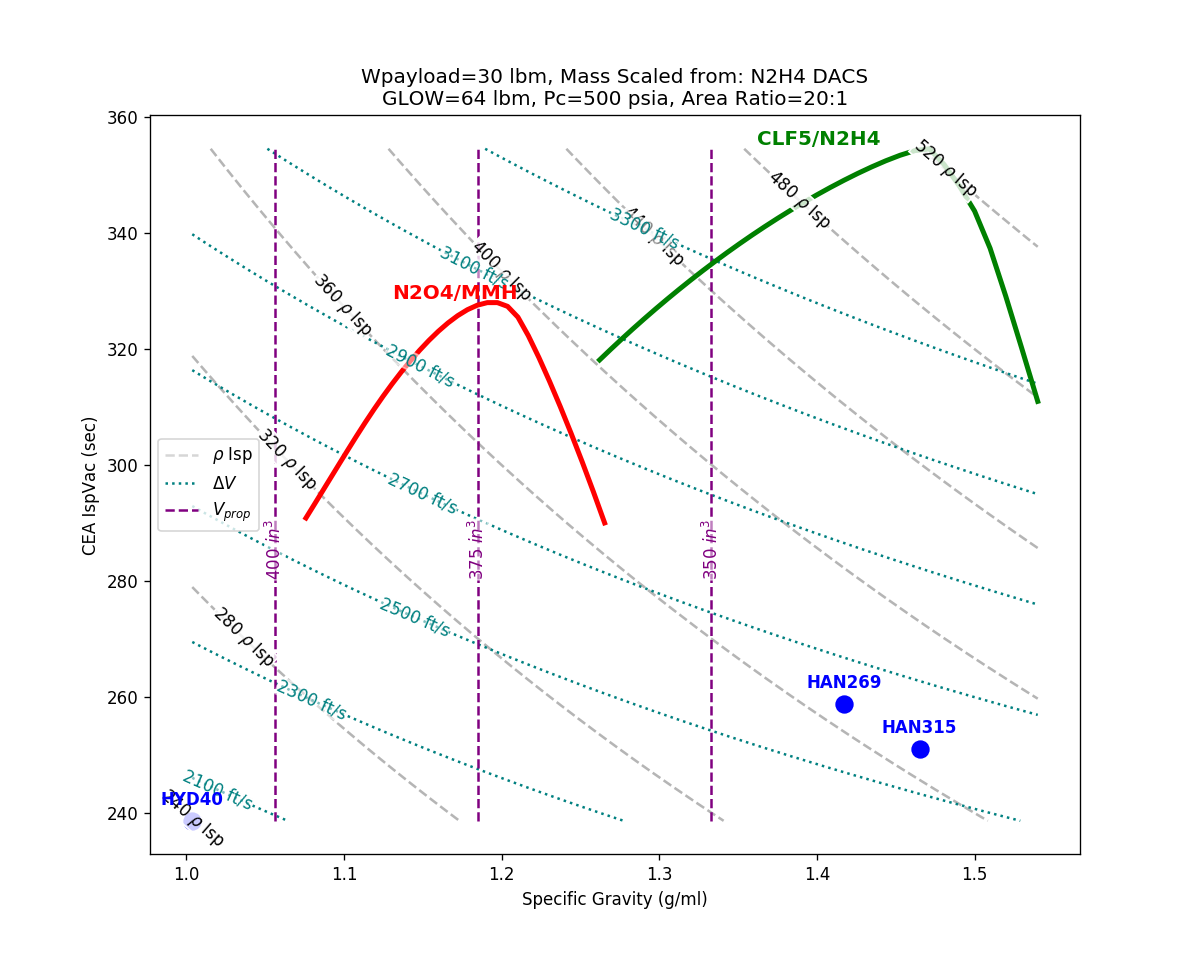Click Image to View Fill Size

The script that created this chart is.

```from rocketcea.biprop_utils.rho_isp_plot_obj import RhoIspPlot
from rocketcea.biprop_utils.veh_stage_obj import ReferenceStage

stg_obj = ReferenceStage(volCuInRef=441.0 , WtInertRef=20.0, WtPayload=30.0,
Name='N2H4 DACS')

rp = RhoIspPlot(bipropL=[('N2O4','MMH'), ('CLF5','N2H4')],
monopropL=['HYD40', 'HAN315', 'HAN269'])